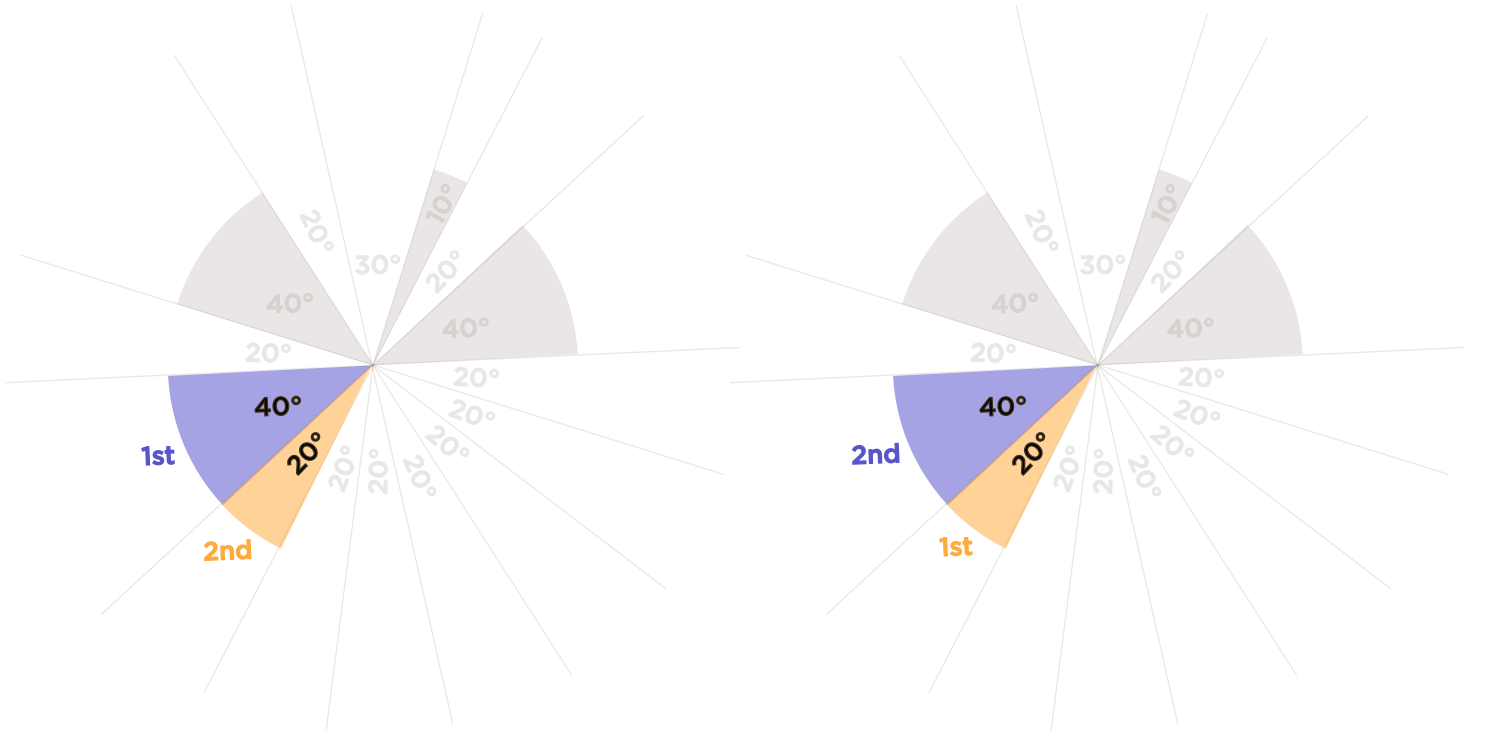# Should same-angles but opposite order combinations be allowed?

• Module 2 Week 2 Day 8 Challenge Part 3

Whether repeat (like same angles but opposite order) combinations are allowed should be specified in mini-question

• @The-Rogue-Blade I don't completely get what you're asking. (assuming you are talking about the opposite order of lines)I think that repeats are not allowed because that would be the same thing. I considered each angle being surrounded by a pair of lines and never thought of the order.• @The-Rogue-Blade I see what you're asking! You're saying that perhaps this pair of angles should be counted twice, depending on which is the first angle and which is the second angle:The double-counting occurs if order matters. For example, in questions like, "How many ways are there to choose two out of five people to stand in a line?"or "How many ways are there to make an ice cream cone with two scoops if you can choose from vanilla, chocolate, mocha, mint chip, or raspberry?"etc., then order matters.

If we say "How many ways are there to choose a pair of students out of 5 to sing in the performance?"then, does order matter? No, it doesn't.

We have a convention of counting "the ways to choose two things out of $$n$$ things," and it's called a "binomial coefficient," which looks like $$\binom{n}{2}$$, or it's called more casually a "choose." You will see more of this in Module 3: Combinatorics.We also saw chooses in Module 0 with the Day 8 lesson about counting the number of triangles out of a bunch of criss-crossing lines. In that lesson, we saw

$$\text{ number of ways to choose } 2 \text{ lines out of } 6 \text{ lines } = \frac{6 \times 5 }{2}$$

We divided by $$2$$ because order didn't matter; whether we chose a line first or second didn't affect the answer.

This mini-question is asking for the number of pairs in the same way, so that's why we don't count the highlighted $$40^{\circ}$$ and $$20^{\circ}$$ angles twice as in the diagram above.• @debbie
That’s getting into combinatorics in a geometry question explanation lol• @RZ923 I wonder if it's as easy to put geometry into a combinatorics problem.......• @debbie
Prof Loh does it in Module 3, Day 4 Challenge Question